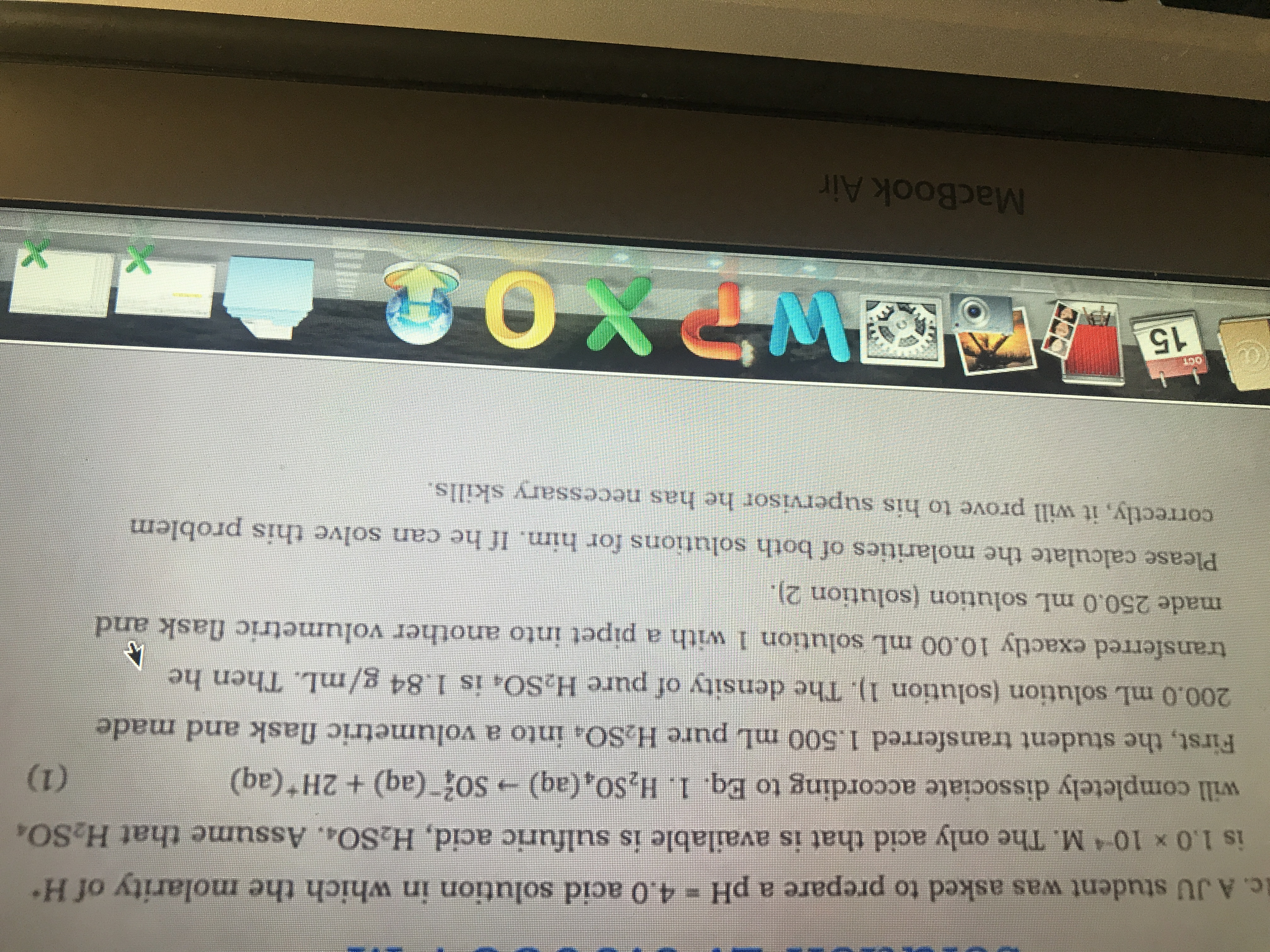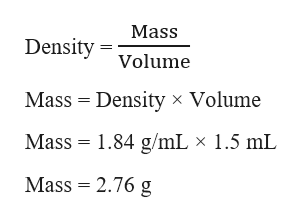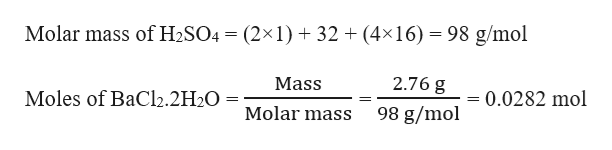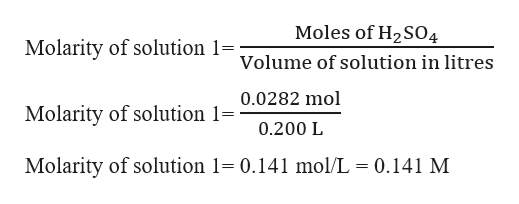# c. A JU student was asked to prepare a pH = 4.0 acid solution in which the molarity of His 1.0 x 104 M. The only acid that is available is sulfuric acid, H2SO4. Assume that H2SOwill completely dissociate according to Eq. 1. H2SO, (aq)So (aq) + 2H (aq)(1)First, the student transferred 1.500 mL pure H.SOa into a volumetric flask andmade200.0 mL solution (solution 1). The density of pure H2sO4 is 1.84 g/mL. Then hetransferred exactly 10.00 mL solution 1 with a pipet into another volumetric flask andmade 250.0 mL solution (solution 2).Please calculate the molarities of both solutions for him. If he can solve this problemcorrectly, it will prove to his supervisor he has necessary skills.WPXO15EFFEESEFMacBook Air

Question
1 viewshelp_outlineImage Transcriptionclosec. A JU student was asked to prepare a pH = 4.0 acid solution in which the molarity of H is 1.0 x 104 M. The only acid that is available is sulfuric acid, H2SO4. Assume that H2SO will completely dissociate according to Eq. 1. H2SO, (aq) So (aq) + 2H (aq) (1) First, the student transferred 1.500 mL pure H.SOa into a volumetric flask and made 200.0 mL solution (solution 1). The density of pure H2sO4 is 1.84 g/mL. Then he transferred exactly 10.00 mL solution 1 with a pipet into another volumetric flask and made 250.0 mL solution (solution 2). Please calculate the molarities of both solutions for him. If he can solve this problem correctly, it will prove to his supervisor he has necessary skills. WPXO 15 EFFEESEF MacBook Air fullscreen
check_circle

star
star
star
star
star
1 Rating
Step 1

Given,

Volume of H2SO4 = 1.500 mL

Volume of solution = 200.0 mL = 0.200 L    (1 mL = 0.001 L)

Density of H2SO4 = 1.84 g/mL

Mass of H2SO4 can be calculated as:help_outlineImage TranscriptioncloseMass Density Volume Mass Density x Volume Mass 1.84 g/mL x 1.5 mL Mass = 2.76 g fullscreen
Step 2

Moles of H2SO4 can be calculated as:help_outlineImage TranscriptioncloseMolar mass of H2SO4 (2x1)32 (4x16) 98 g/mol 2.76 g Mass Moles of BaCl2.2H2O - = 0.0282 mol Molar mass 98 g/mol fullscreen
Step 3

Molarity of solution 1 can...help_outlineImage TranscriptioncloseMoles of H2SO4 Molarity of solution 1= Volume of solution in litres 0.0282 mol Molarity of solution 1= 0.200 L Molarity of solution 1 0.141 mol/L = 0.141 M fullscreen

### Want to see the full answer?

See Solution

#### Want to see this answer and more?

Solutions are written by subject experts who are available 24/7. Questions are typically answered within 1 hour.*

See Solution
*Response times may vary by subject and question.
Tagged in

### Solutions